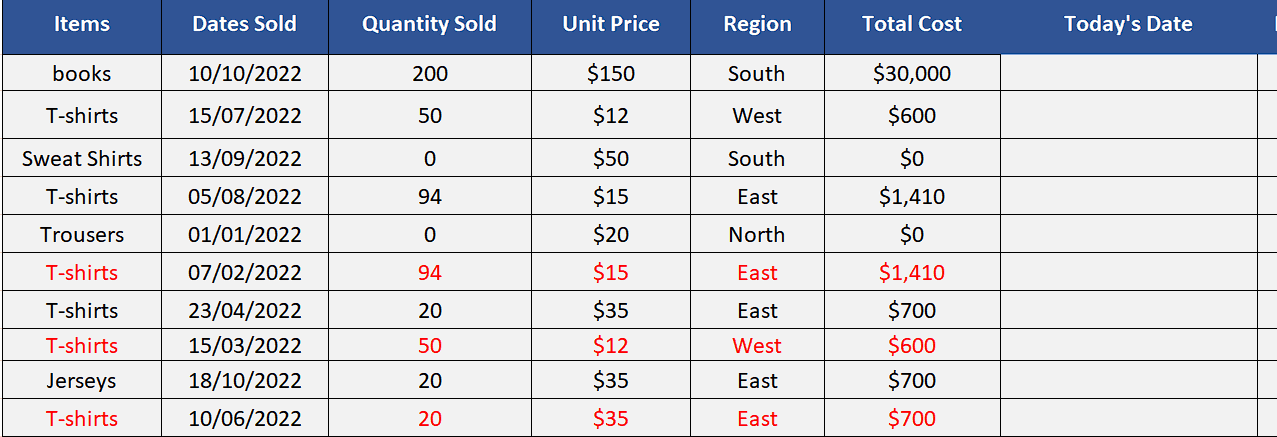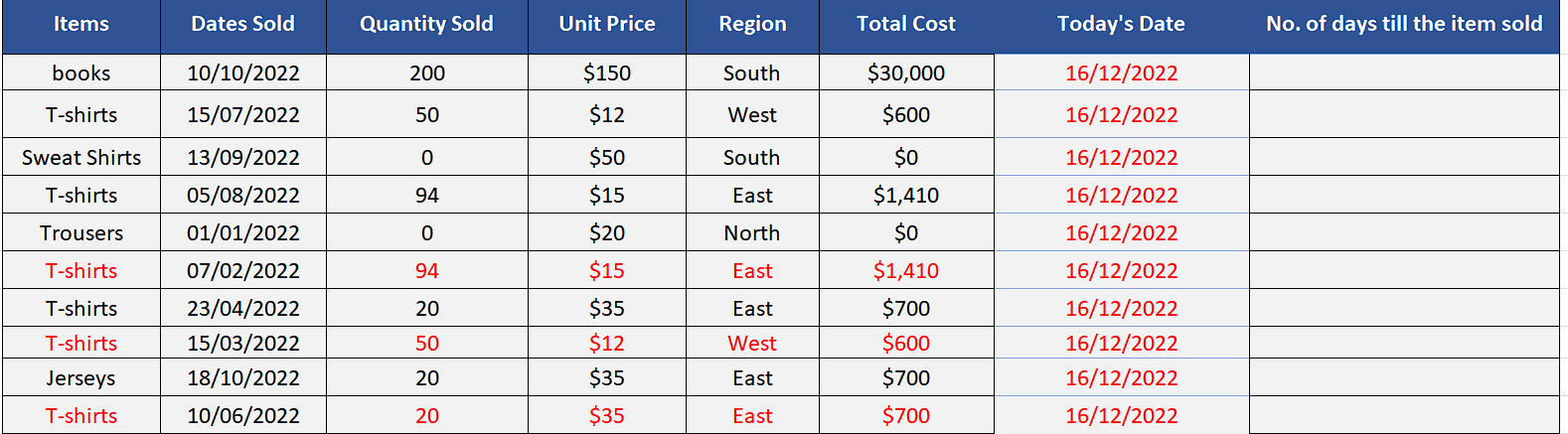# How to use the shortcut keys for the date in Excel

You can also enter dates by using the shortcuts in Excel. However, these shortcuts only let you enter the current date i.e., today’s date in any cell. In this tutorial we’ll learn what is the shortcut for date in Excel and how to use it.

The current date in Excel can be useful in a number of ways. One common use of the current date is to automatically update a worksheet or report with the most up-to-date information. For example, you might use the current date to show the date that a report was generated, or to track the number of days that have passed since a particular event. We are going to do the same with our dataset shown above. We’ll calculate the no. of days passed since the items are sold.

Let’s see what is the shortcut for entering the current date in Excel in the following steps.Dates in Excel can be represented using a variety of formats, such as “01/01/2022” for January 1, 2022, or “January 1, 2022” for the same date. Excel will automatically recognize and convert these dates to the appropriate serial number.

### Step – 1 Select the cell and use the shortcut key– The shortcut key for entering the current date is CTRL+; (semicolon). So, select the cell H3 and use this shortcut key. It will enter the current date in this cell.
– Now hold on the CTRL key again and use the fill handle to copy the value of the current date till the end of the data range as shown above.
– If we wish to enter the time with the date as well then, we can use CTRL+SHIFT+;.

### Step 2 – Calculate the difference of days– To calculate the difference of the days since the items are sold and today, we will have to simply subtract the current date from the date the item was sold. We’ll use the following formula,
=H3 – C3

– If we want to make the sheet dynamic then we’ll have to use the TODAY function in H3. That will make the calculations dynamic and whenever we’ll open the sheet the calculation will be updated according to today as shown above.